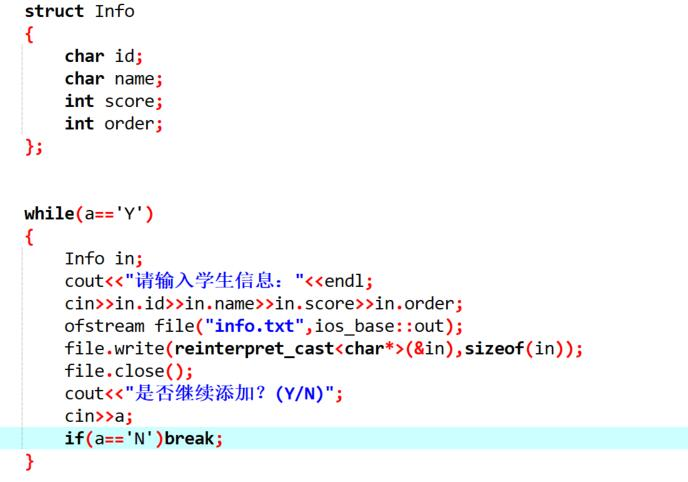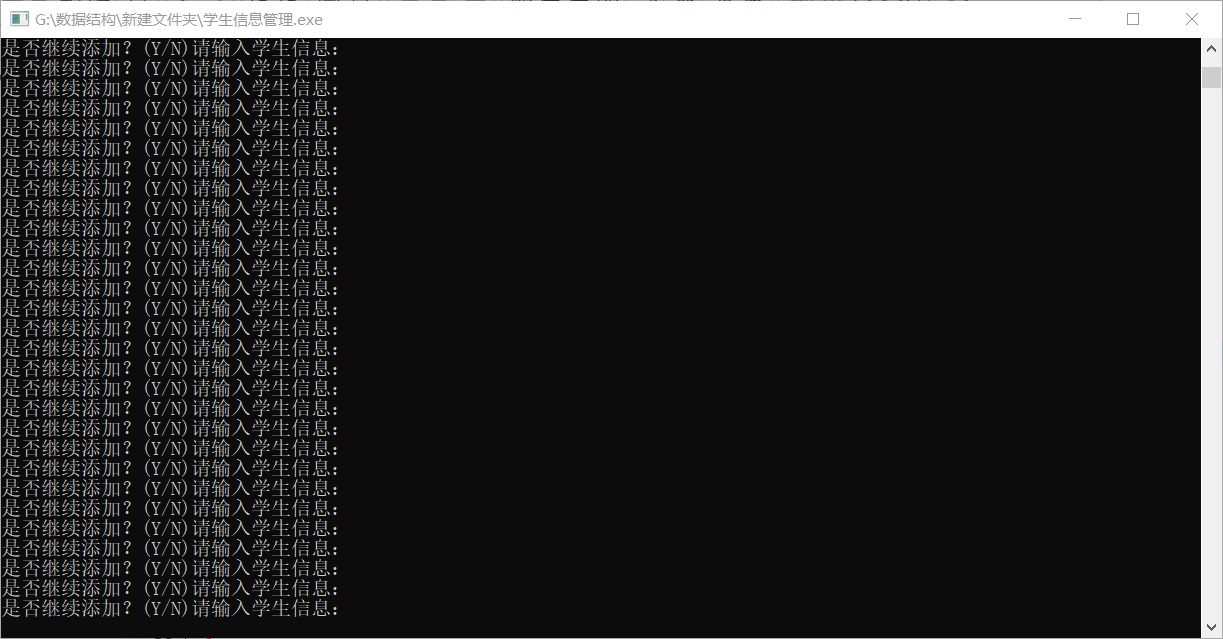c++循环语句while中为什么无法使用cin>>语句进行循环输入？2个回答c++循环语句while中为什么无法使用cin>>语句进行循环输入？

c++do while语句死循环
#include<iostream> using namespace std; int main() { do { double income, tax; cout << "enter the income:"; if (!(cin >> income) || income < 0) { cout << "请输入正数数字!"; cin.clear(); continue; } else if (income<=5000) { tax = 0; } else if (income<=15000) { tax = (income - 5000 )* 0.1; } else if (income <= 35000) { tax = (income - 15000)* 0.15+1000; } else { tax = (income - 35000)* 0.2 + 4000; } cout << tax<<endl; } while (true); cin.get(); cin.get(); return 0; } 输入数字的话会没有事，可以循环下去。但是输入字母，就不会管下一次的cin，一直循环，不知道为什么

#include <iostream.h> main() { int i; while(i!=-1) { cout<<"i=" cin>>i; cout<<endl; } } 上面的程序，如果输入的不合法，比如输入一个字符，那就将陷入死循环。解决的办法 有个一，把cin>>i;语句移到判断循环的语句，那么输入的如果是不合法的变量，他将跳出循环 。
C++求前n个素数之和（循环语句的问题）？

while(cin>>value)语句以后输入跳过
while(cin>>value) {a+=value;} cout<<a<<endl; int b; cin>>b; return 0; 为什么在用ctrl+z后回车结束第一个cin的输入后程序不在进行b的输入

#include <iostream> #include <cstdlib> int main() { using namespace std; const int arsize= 7; char name[arsize]; char dessert [arsize]; int i=0; do{ i++; cout<<"Enter your name:\n"; cin.get(name,arsize); if(i>=2) cin.get(); cout<<"Enter your favorite dessert: \n"; cin.get(dessert,arsize); cout<<"Now I have some delicious|"<<dessert<<"|for you ,"<<name<<" .\n"; }while(i<=arsize); system("pause"); /* ************** cin.getline(name,20); cout<<"Enter your favorite dessert:\n"; cin.getline(dessert,20); cout<<"Now I have some "<<dessert<<" for "<<name<<endl; *********************/ return 0; } 程序测试结果如图 ![图片说明](https://img-ask.csdn.net/upload/201711/27/1511786484_148963.png) ![图片说明](https://img-ask.csdn.net/upload/201711/27/1511786505_990048.png)

C++中cctype头文件中的isdigit()函数如果参数是0~9，则函数返回true，这个函数只能判断是个数么？如果我要判断两位数、三位数......还能用么？ while（cin>>num） 其中num为整形变量，其中括号中的语句可以判断输入是不是数字？是怎么样的原理？ 还有其他方法么？ASCII怎么用呢？
C++while语句问题,中间的条件区分是不是可以区分变量的类型
while (cin >> rplace.x >> rplace.y) 内部赋予的是double变量类型，是不是输入char类型就会提示false 新手刚开始学习C++

![图片说明](https://img-ask.csdn.net/upload/201910/18/1571404065_293549.jpg) ``` #include <iostream> using namespace std; int main() { double n,max,min; while(cin>>n) { if(n>=max) {max=n; cout<<max<<"the largest so far"<<endl;} if(n<=min) {min=n; cout<<min<<"the smallest so far"<<endl;} if(n<=max&&n>=min) cout<<n<<endl; } return 0; } ``` 这里输入的第一个数怎么处理
Xcode下输入vector1回车或者ctrl+D就return 0 ，无法输入vector2 ，语句中cin.clear()好像没起到作用？
#include <iostream> #include <vector> using namespace std; int main() { vector<int> ivec1, ivec2; int ival1, ival2; cout << "enter numbers for vector 1 : " << endl; while (cin >> ival1) { ivec1.push_back(ival1); } cin.clear(); cout << "enter numbers for vector 2 : " << endl; while (cin >> ival2) { ivec2.push_back(ival2); }
C++编程问题请大神赐教谢谢

#include <iostream> #include <cmath> using namespace std; void calccuboid(); void calccylinder(); void calccone(); int main() { int choice = -1; while (choice) { cout <<"1.长方体"<<endl; cout <<"2.圆柱体"<<endl; cout <<"3.圆锥体"<<endl; cin >> choice; switch (choice) { case 1: calccuboid(); break; case 2: calccylinder(); break; case 3: calccone(); break; } } cout <<"感谢使用本软件！" <<endl; } void calccuboid() { double len,width,height; cout <<"请输入长宽高："; cin >>len>>width>>height; double v = len*width*height; cout <<"长方体的体积为："<<v<<endl; } void calccylinder() { double radius,height; cout <<"请输入半径和高："; cin >>radius>>height; double v = 3.14*pow(radius,2)*height; cout <<"圆柱体的体积为："<<v<<endl; } void calccone() { double radius,height; cout <<"请输入半径和高："; cin >>radius>>height; double v = 3.14/3*pow(radius,2)*height; cout <<"圆锥体的体积为："<<v<<endl; } 为什么输入0，就会出现cout的感谢使用本软件！语句而输入4，5之类的只会继续循环 。
C++ 两数之间的所有整数
#include <iostream> using namespace std; int main() { int i = 1; while (i == 1) { int num1, num2; cout << "Please input two numbers" << endl; cin >> num1 >> num2; if (num1 <= num2) { int i = num1; while (i >= num1 && i <= num2)//&&逻辑与 只要一个不为真就是假的； { cout << i << endl; i++; } } else { int i = num2; while (i >= num2 && i <= num1) { //这里用到了判断语句if else 判断 num1是大于num2，还是num1小于num2的判断语句 cout << i << endl; i++; } } } return 0; } 麻烦用for，来改写这段代码 麻烦有知道的 帮忙改写一下

while(cin>>index0>>str>>index1) 我有点不理解，希望帮帮忙，谢谢

``` #include <iostream> #include <string> using namespace std; void strcount(const string); int main() { string input; cout<<"enter a line:"<<endl; cin>>input; while(!input.empty()) { while(cin.get()!='\n') continue; strcount(input); cout<<"enter next line (empty line to quit):"<<endl; cin>>input; } cout<<"bye"<<endl; return 0; } void strcount(const string str) { static int total=0; int count=0; cout<<"\""<<str<<"\"contains "; count=str.length(); total+=count; cout<<count<<"characters"<<endl; cout<<total<<"characters total"<<endl; } ``` 如何能用简短的语句达到输入为空就跳出循环呢...执行完一次以后我敲回车只是光标换行，如何能将string类的对象设置为空？我用C-free和DEV都试过了都是这个样子![图片说明](https://img-ask.csdn.net/upload/201812/31/1546237040_816862.png)

#include<iostream> #include<cstdlib> #include<cmath> #include<vector> using namespace std; void Prime() { int k; vector<int> dv1; vector<int> dv2; vector<int>::iterator n; vector<int>::iterator m; do{ cout << "请输入范围限定值c:" << " "; cin >> k; if (!cin >> k) { cout << "输入无效，请输入正确的数据！" << endl; continue; } for (int i = 1; i <= k; ++i) { int j = 2; for (; j <= sqrt(i); ++j) { if (i%j == 0)//用平方根以内的数去除，如果能除尽，则说明是合数，则跳出此次循环，此时i加1 //如果j自增到平方根还不能除尽，则j加1，说明是质数，此时j=sqrt(i)+1，自动跳出本循环 break; } if (j > sqrt(i) && i != 1) //j=sqrt(i)+1时说明i为质数，符合这个if条件，执行输出语句 dv1.push_back(i); //cout << j <<endl;此为测试j值语句 else if (j <= sqrt(i) && i != 1) dv2.push_back(i); } cout << "质数为：" << " "; for (n = dv1.begin(); n != dv1.end(); n++) cout << *n << " "; cout << endl; cout << "合数为：" << " "; for (m = dv2.begin(); m != dv2.end(); m++) cout << *m << " "; cout << endl; dv1.clear(); dv2.clear(); } while (cin >> k); } void main() { Prime(); } 不通过反复系统调用，而是直接压缩到一个函数内解决，错误程序如下，求帮忙改正一下，谢谢！

#include<iostream> using namespace std; void hanoi(int n,char a,char b,char c) { if(n==1) cout<<n<<" "<<a<<"->"<<c<<endl; else{ hanoi(n-1,a,c,b); cout<<n<<" "<<a<<"->"<<c<<endl;//指这条代码 hanoi(n-1,b,a,c); } } int main() { int n; while(cin>>n) hanoi(n,'A','B','C'); return 0; } 请问为什么那条cout输出语句（代码中注释部分）为什么在中间 而不是在hanoi(n-1,b,a,c);后面或者有两个语句后面都cout。 谢谢~

Devc++编译器中用c写文件存取为什么打开文件为乱码？
//首先我写了学生信息管理系统的添加数据函数，需要将数据保存在磁盘里，将数据写入文件那一块用的c语言，其他为c++，代码如下： void Add() { char a='Y'; FILE *fp; if((fp=fopen("informations.dat","w"))==NULL) { printf("无法打开此文件 ！\n"); exit(0); } while(a=='Y') { //if() Info in; cout<<"请输入学生信息："<<endl; cin>>in.id>>in.name>>in.score>>in.order; if(fwrite(&in,sizeof(struct Info),1,fp)!=1) cout<<"file write error"<<endl; cout<<"是否继续添加？(Y/N)"; cin>>a; //printf("%s",in.id); } fclose(fp); putchar(10); } //此时打开文件里是乱码，如下图 ![图片说明](https://img-ask.csdn.net/upload/201812/14/1544725957_932678.png) //另外写了一个只用来存文件的代码用来测试，打开就不是乱码，是正常数据。代码如下 ``` #include<iostream> using namespace std; struct student { string name; int grade; }; int main() { FILE *fp; student stu; if((fp=fopen("information","w"))==NULL) { printf("无法打开此文件 ！\n"); exit(0); } printf("请输入学号："); scanf("%s",&stu.name); printf("请输入成绩："); scanf("%s",&stu.grade); if(fwrite(&stu,sizeof(struct student),1,fp)!=1) cout<<"file write error"<<endl; fclose(fp); putchar(10); return 0; } ``` //若是将add函数中的信息输入语句cin 用scanf替换则文件中存不进数据，代码如下 ``` void Add() { char a='Y'; FILE *fp; //ofstream file("info.xlsx",ios_base::in); initial(); if((fp=fopen("informations.dat","w"))==NULL) { printf("无法打开此文件 ！\n"); exit(0); } while(a=='Y') { //if() Info in; cout<<"请输入学生信息："<<endl; //cin>>in.id>>in.name>>in.score>>in.order; scanf("%s",&in.id); printf("请输入姓名："); scanf("%s",&in.name); printf("请输入成绩："); scanf("%d",&in.score); printf("请输入排名："); scanf("%d",&in.order); if(fwrite(&in,sizeof(struct Info),1,fp)!=1) cout<<"file write error"<<endl; cout<<"是否继续添加？(Y/N)"; cin>>a; //printf("%s",in.id); } fclose(fp); putchar(10); } ``` 求解，很想知道为什么

Java学习的正确打开方式

linux系列之常用运维命令整理笔录

Python十大装B语法
Python 是一种代表简单思想的语言，其语法相对简单，很容易上手。不过，如果就此小视 Python 语法的精妙和深邃，那就大错特错了。本文精心筛选了最能展现 Python 语法之精妙的十个知识点，并附上详细的实例代码。如能在实战中融会贯通、灵活使用，必将使代码更为精炼、高效，同时也会极大提升代码B格，使之看上去更老练，读起来更优雅。

Docker 技术发展为微服务落地提供了更加便利的环境，使用 Docker 部署 Spring Boot 其实非常简单，这篇文章我们就来简单学习下。首先构建一个简单的 S...

SQL-小白最佳入门sql查询一

redis分布式锁，面试官请随便问，我都会

Nginx 原理和架构
Nginx 是一个免费的，开源的，高性能的 HTTP 服务器和反向代理，以及 IMAP / POP3 代理服务器。Nginx 以其高性能，稳定性，丰富的功能，简单的配置和低资源消耗而闻名。 Nginx 的整体架构 Nginx 里有一个 master 进程和多个 worker 进程。master 进程并不处理网络请求，主要负责调度工作进程：加载配置、启动工作进程及非停升级。worker 进程负责处...
“狗屁不通文章生成器”登顶GitHub热榜，分分钟写出万字形式主义大作

《程序人生》系列-这个程序员只用了20行代码就拿了冠军

11月8日，由中国信息通信研究院、中国通信标准化协会、中国互联网协会、可信区块链推进计划联合主办，科技行者协办的2019可信区块链峰会将在北京悠唐皇冠假日酒店开幕。 　　区块链技术被认为是继蒸汽机、电力、互联网之后，下一代颠覆性的核心技术。如果说蒸汽机释放了人类的生产力，电力解决了人类基本的生活需求，互联网彻底改变了信息传递的方式，区块链作为构造信任的技术有重要的价值。 　　1...
Java世界最常用的工具类库
Apache Commons Apache Commons有很多子项目 Google Guava 参考博客

【技巧总结】位运算装逼指南

Android 9.0 init 启动流程